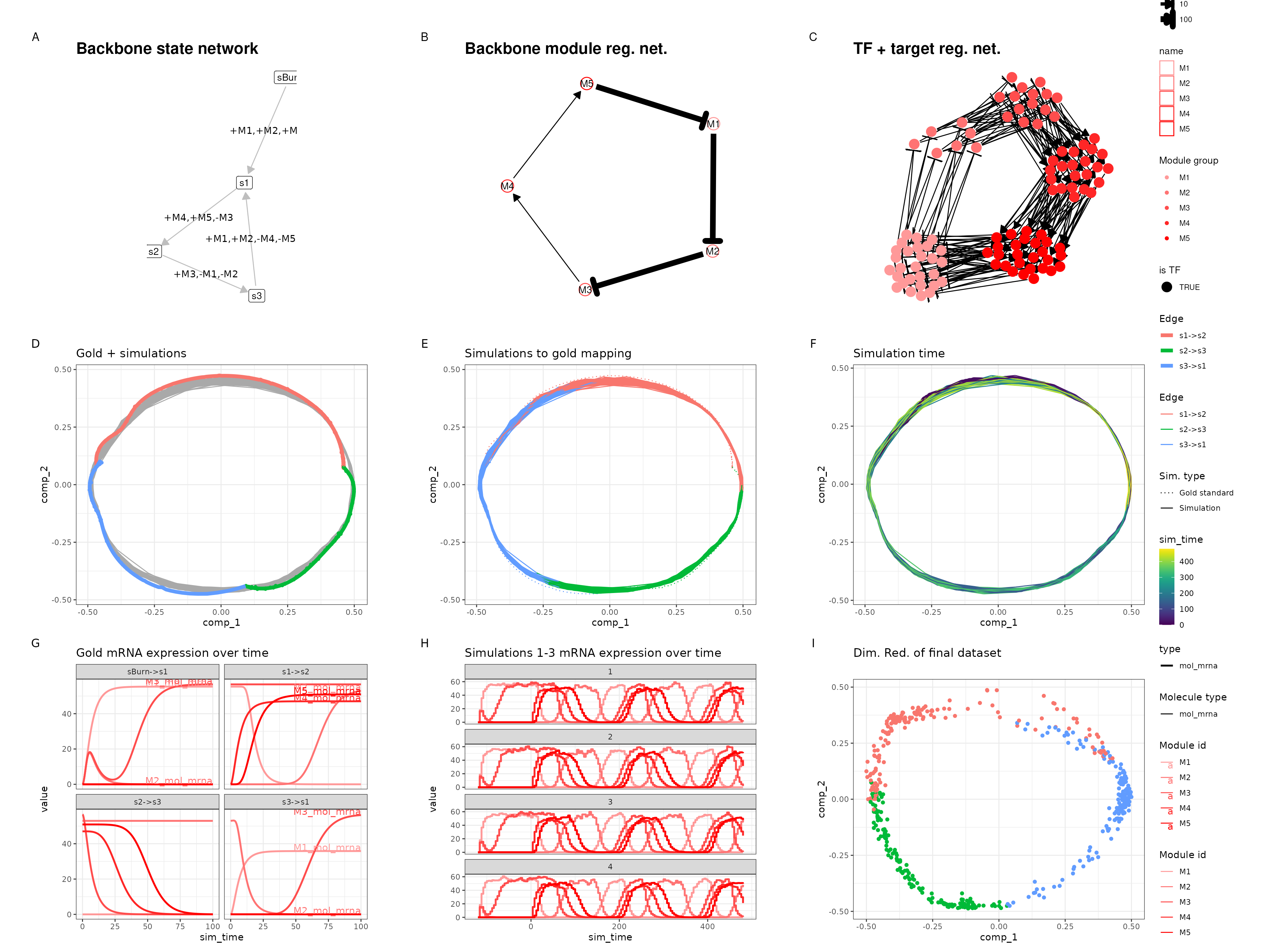library(dyngen)

This vignette demonstrates the different dynamic processes topologies (e.g. bifurcating and cyclic). Note that, for the sake of reducing runtime for generating this vignette, the simulations are ran with reduced settings. Check out the vignette on tweaking parameters for information on how the different parameters are changed.

You can find a full list of backbones using ?list_backbones. This vignette will showcase each of them individually.

## Linear

set.seed(1)

backbone <- backbone_linear()

init <- initialise_model(
backbone = backbone,
num_cells = 500,
num_tfs = 100,
num_targets = 0,
num_hks = 0,
simulation_params = simulation_default(census_interval = 10, ssa_algorithm = ssa_etl(tau = 300 / 3600)),
verbose = FALSE
)
out <- generate_dataset(init, make_plots = TRUE)
out$plot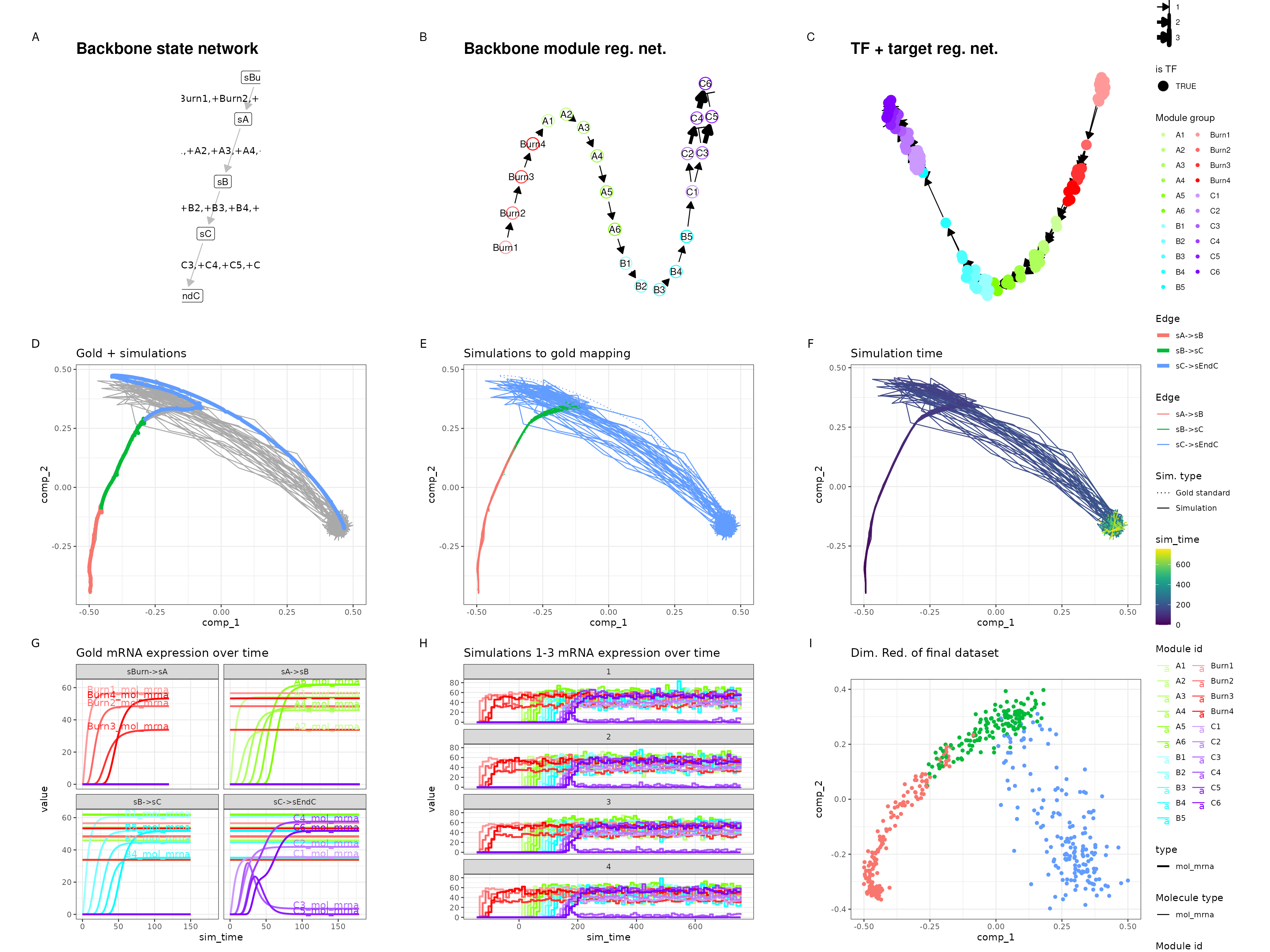## Bifurcating set.seed(2) backbone <- backbone_bifurcating() init <- initialise_model( backbone = backbone, num_cells = 500, num_tfs = 100, num_targets = 0, num_hks = 0, simulation_params = simulation_default(census_interval = 10, ssa_algorithm = ssa_etl(tau = 300 / 3600)), verbose = FALSE ) out <- generate_dataset(init, make_plots = TRUE) out$plot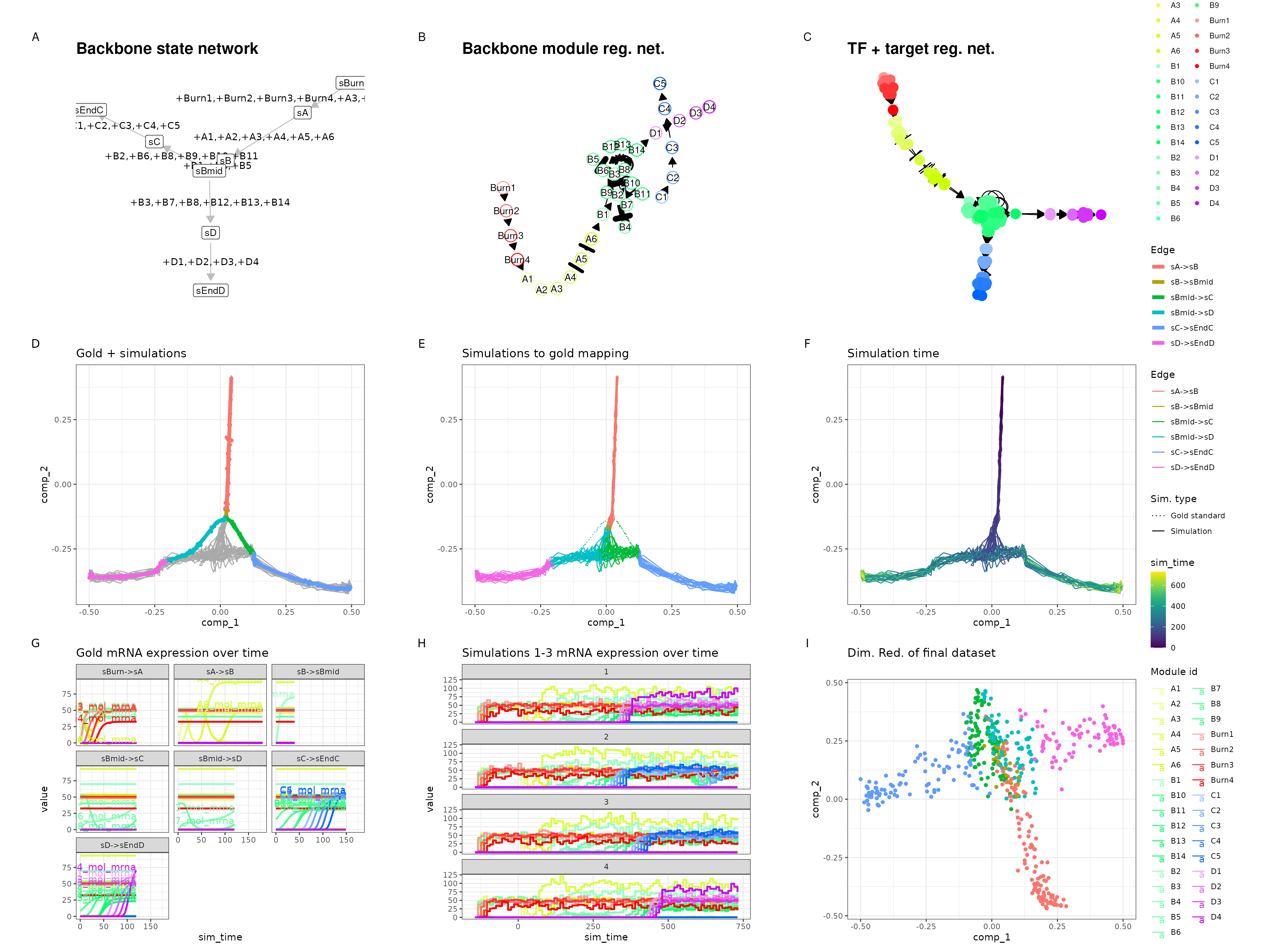## Bifurcating converging

set.seed(3)

backbone <- backbone_bifurcating_converging()

init <- initialise_model(
backbone = backbone,
num_cells = 500,
num_tfs = 100,
num_targets = 0,
num_hks = 0,
simulation_params = simulation_default(census_interval = 10, ssa_algorithm = ssa_etl(tau = 300 / 3600)),
verbose = FALSE
)
out <- generate_dataset(init, make_plots = TRUE)
#> Warning in params$fun(network = network, sim_meta = sim_meta, params = model$experiment_params, : One of the branches did not obtain enough simulation steps.
#>   Increase the number of simulations (see ?simulation_default) or decreasing the census interval (see ?simulation_default).
out$plot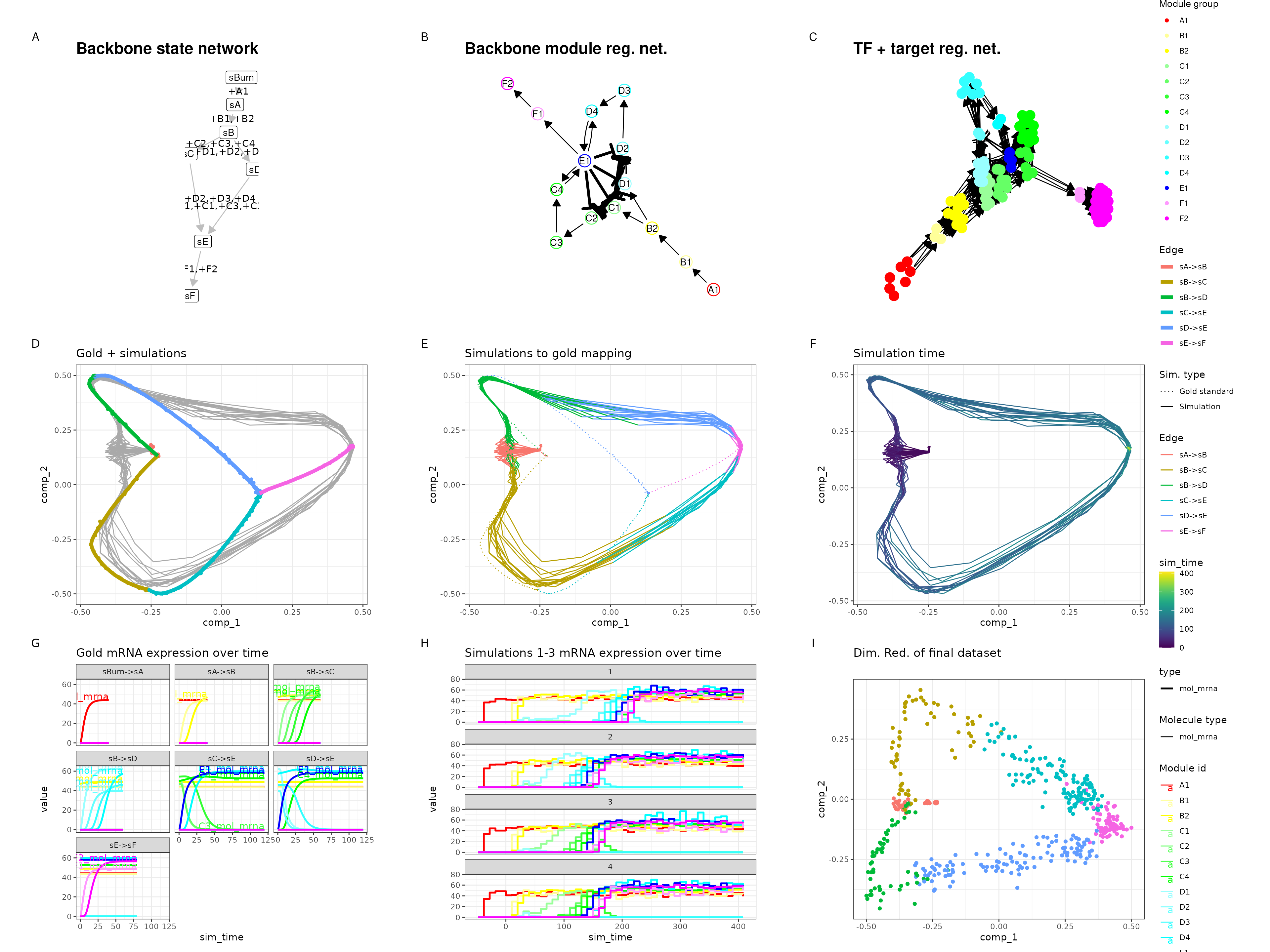## Bifurcating cycle set.seed(4) backbone <- backbone_bifurcating_cycle() init <- initialise_model( backbone = backbone, num_cells = 500, num_tfs = 100, num_targets = 0, num_hks = 0, simulation_params = simulation_default(census_interval = 10, ssa_algorithm = ssa_etl(tau = 300 / 3600)), verbose = FALSE ) out <- generate_dataset(init, make_plots = TRUE) #> Warning in params$fun(network = network, sim_meta = sim_meta, params = model$experiment_params, : One of the branches did not obtain enough simulation steps. #> Increase the number of simulations (see ?simulation_default) or decreasing the census interval (see ?simulation_default). out$plot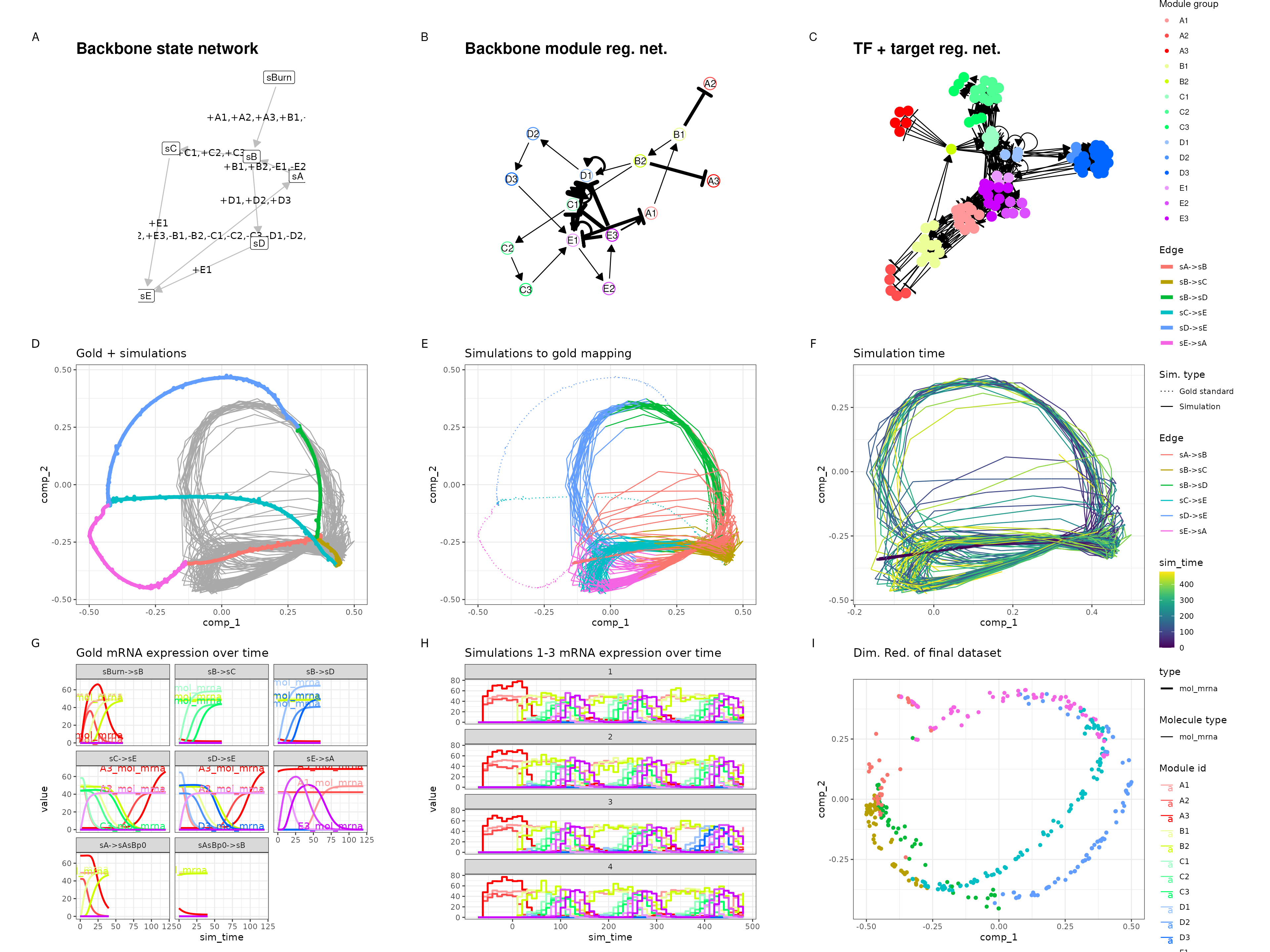## Bifurcating loop

set.seed(5)

backbone <- backbone_bifurcating_loop()

init <- initialise_model(
backbone = backbone,
num_cells = 500,
num_tfs = 100,
num_targets = 0,
num_hks = 0,
simulation_params = simulation_default(census_interval = 10, ssa_algorithm = ssa_etl(tau = 300 / 3600)),
verbose = FALSE
)
out <- generate_dataset(init, make_plots = TRUE)
#> Warning in params$fun(network = network, sim_meta = sim_meta, params = model$experiment_params, : One of the branches did not obtain enough simulation steps.
#>   Increase the number of simulations (see ?simulation_default) or decreasing the census interval (see ?simulation_default).
out$plot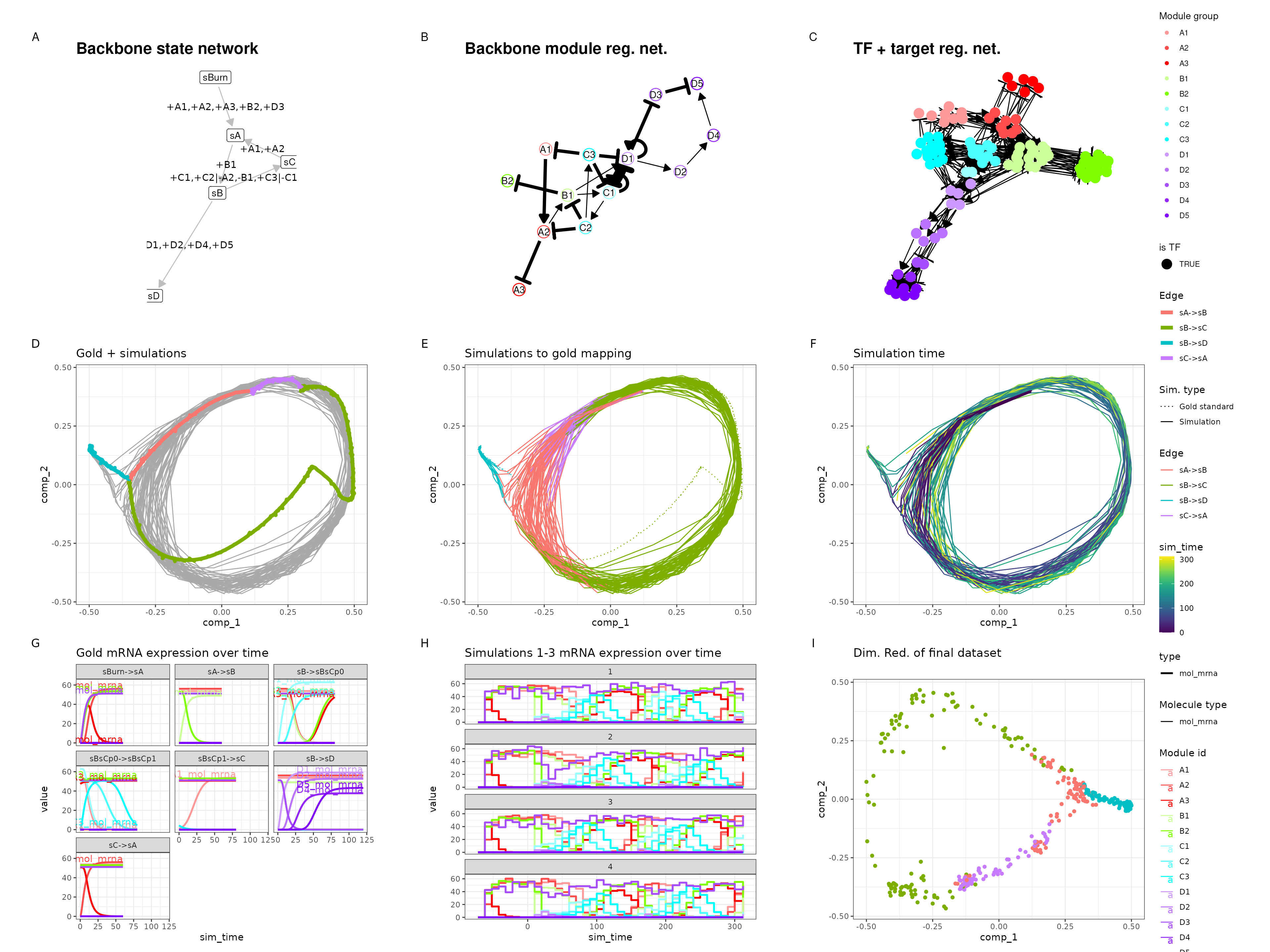## Binary tree set.seed(6) backbone <- backbone_binary_tree( num_modifications = 2 ) init <- initialise_model( backbone = backbone, num_cells = 500, num_tfs = 100, num_targets = 0, num_hks = 0, simulation_params = simulation_default(census_interval = 10, ssa_algorithm = ssa_etl(tau = 300 / 3600)), verbose = FALSE ) out <- generate_dataset(init, make_plots = TRUE) out$plot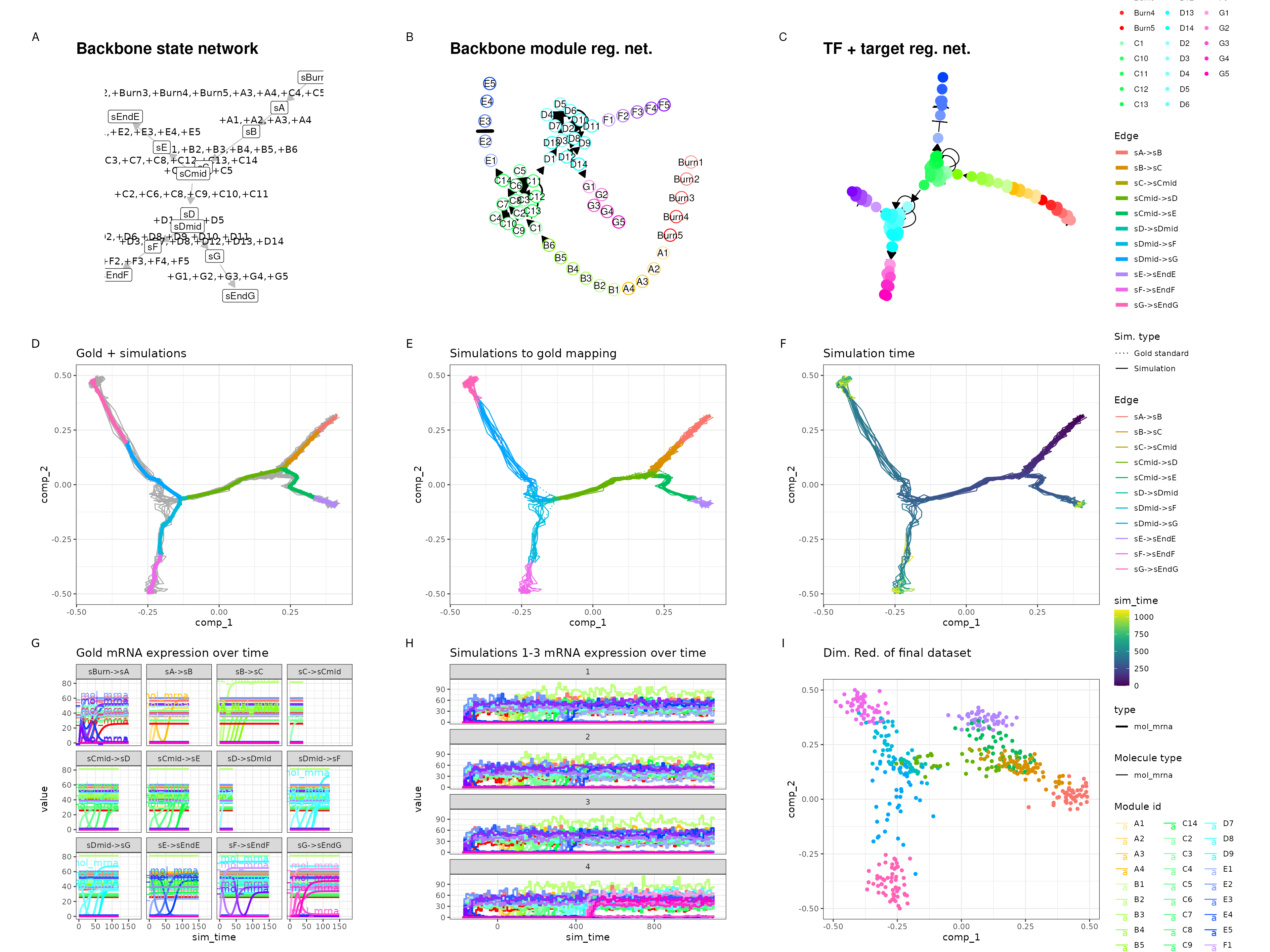## Branching

set.seed(7)

backbone <- backbone_branching(
num_modifications = 2,
min_degree = 3,
max_degree = 3
)

init <- initialise_model(
backbone = backbone,
num_cells = 500,
num_tfs = 100,
num_targets = 0,
num_hks = 0,
simulation_params = simulation_default(census_interval = 10, ssa_algorithm = ssa_etl(tau = 300 / 3600)),
verbose = FALSE
)
out <- generate_dataset(init, make_plots = TRUE)
#> Warning in .generate_cells_predict_state(model): Simulation does not contain all
#> gold standard edges. This simulation likely suffers from bad kinetics; choose a
#> different seed and rerun.
#> Warning in params$fun(network = network, sim_meta = sim_meta, params = model$experiment_params, : Certain backbone segments are not covered by any of the simulations. If this is intentional, please ignore this warning.
#>   Otherwise, increase the number of simulations (see ?simulation_default) or decreasing the census interval (see ?simulation_default).
#> Warning in params$fun(network = network, sim_meta = sim_meta, params = model$experiment_params, : One of the branches did not obtain enough simulation steps.
#>   Increase the number of simulations (see ?simulation_default) or decreasing the census interval (see ?simulation_default).
out$plot## Consecutive bifurcating set.seed(8) backbone <- backbone_consecutive_bifurcating() init <- initialise_model( backbone = backbone, num_cells = 500, num_tfs = 100, num_targets = 0, num_hks = 0, simulation_params = simulation_default(census_interval = 10, ssa_algorithm = ssa_etl(tau = 300 / 3600)), verbose = FALSE ) out <- generate_dataset(init, make_plots = TRUE) #> Warning in .generate_cells_predict_state(model): Simulation does not contain all #> gold standard edges. This simulation likely suffers from bad kinetics; choose a #> different seed and rerun. #> Warning in params$fun(network = network, sim_meta = sim_meta, params = model$experiment_params, : Certain backbone segments are not covered by any of the simulations. If this is intentional, please ignore this warning. #> Otherwise, increase the number of simulations (see ?simulation_default) or decreasing the census interval (see ?simulation_default). #> Warning in params$fun(network = network, sim_meta = sim_meta, params = model$experiment_params, : One of the branches did not obtain enough simulation steps. #> Increase the number of simulations (see ?simulation_default) or decreasing the census interval (see ?simulation_default). out$plot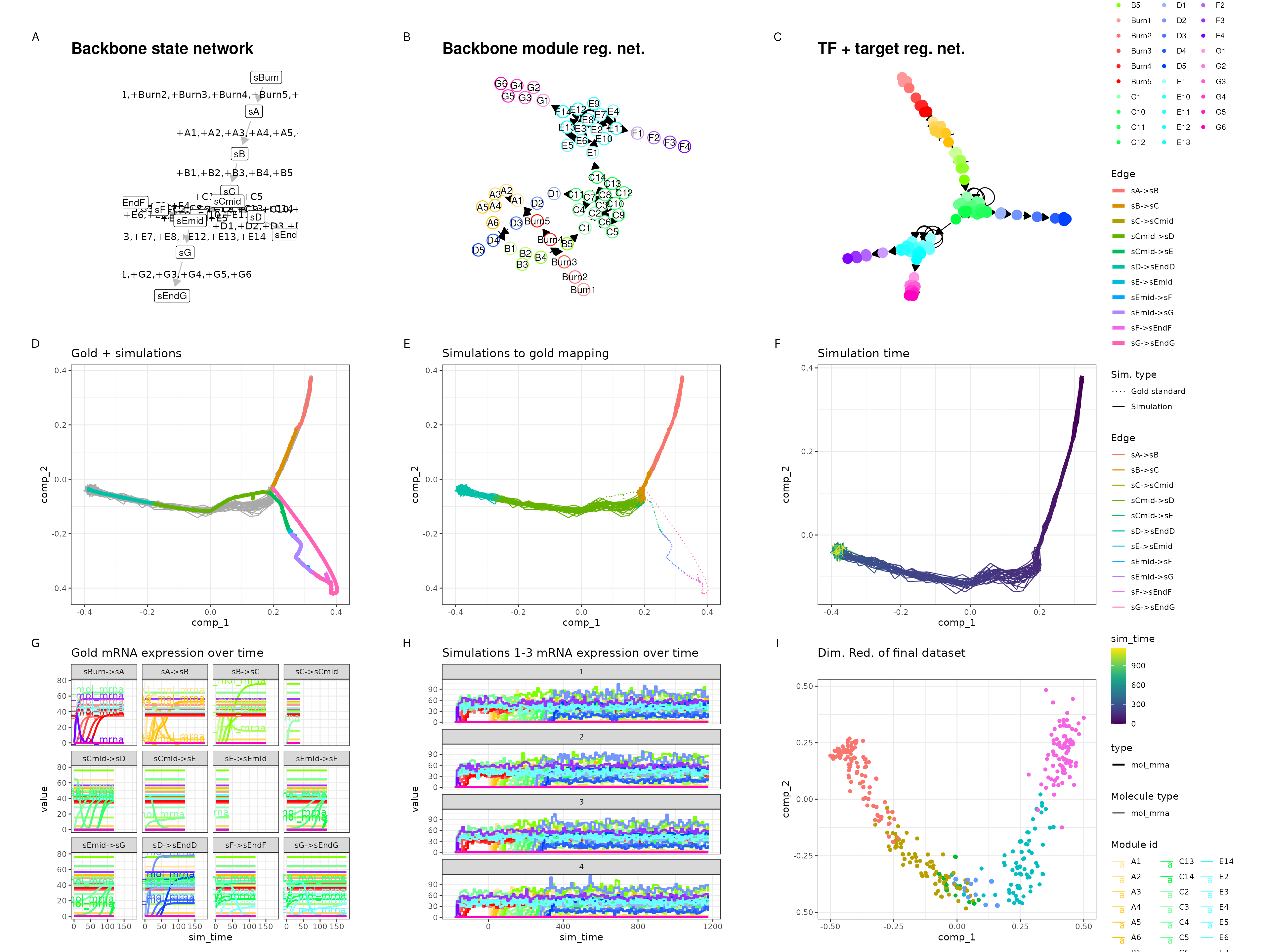## Trifurcating

set.seed(9)

backbone <- backbone_trifurcating()

init <- initialise_model(
backbone = backbone,
num_cells = 500,
num_tfs = 100,
num_targets = 0,
num_hks = 0,
verbose = FALSE
)
out <- generate_dataset(init, make_plots = TRUE)
out$plot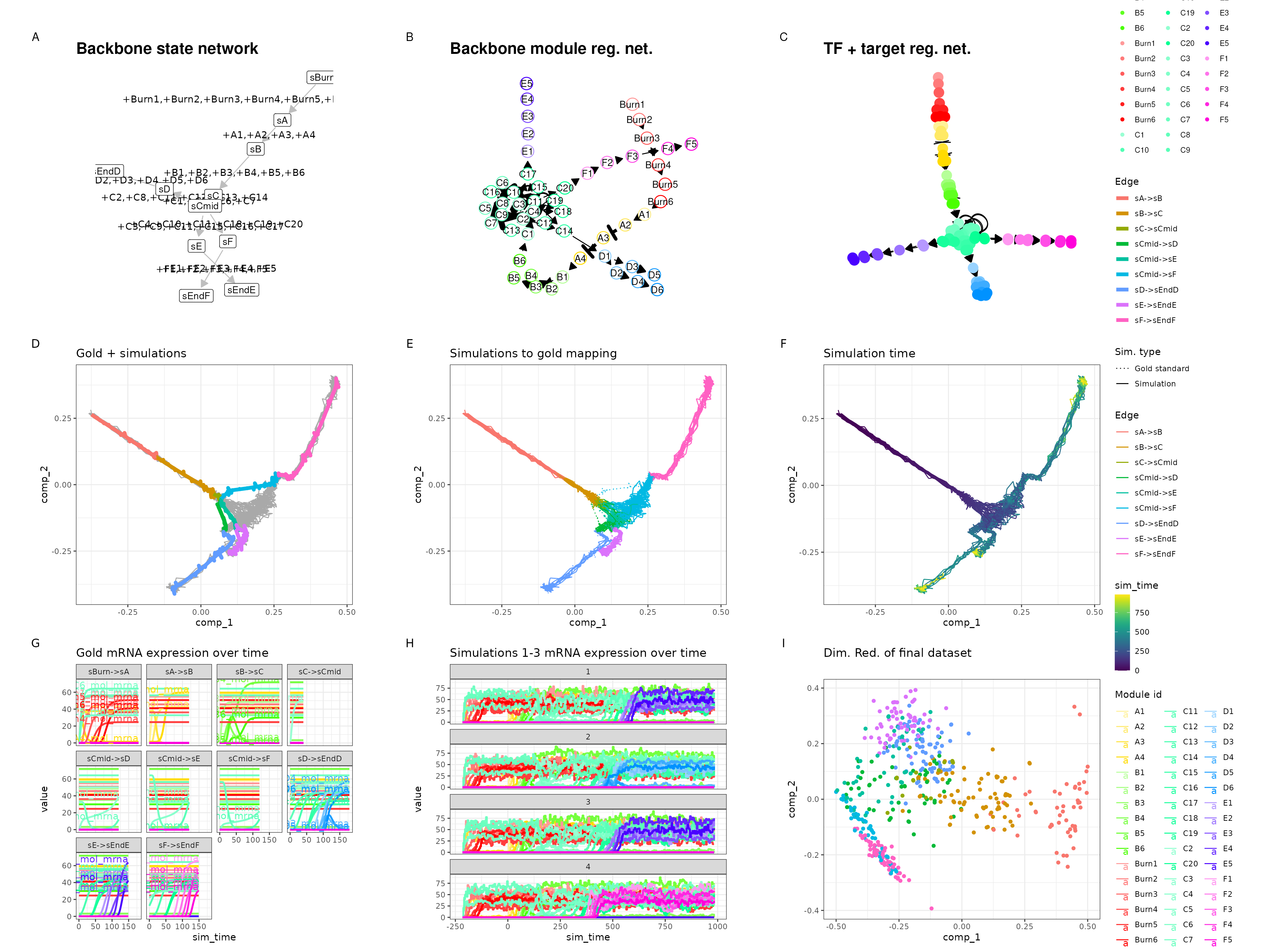## Converging set.seed(10) backbone <- backbone_converging() init <- initialise_model( backbone = backbone, num_cells = 500, num_tfs = 100, num_targets = 0, num_hks = 0, simulation_params = simulation_default(census_interval = 10, ssa_algorithm = ssa_etl(tau = 300 / 3600)), verbose = FALSE ) out <- generate_dataset(init, make_plots = TRUE) #> Warning in params$fun(network = network, sim_meta = sim_meta, params = model$experiment_params, : One of the branches did not obtain enough simulation steps. #> Increase the number of simulations (see ?simulation_default) or decreasing the census interval (see ?simulation_default). out$plot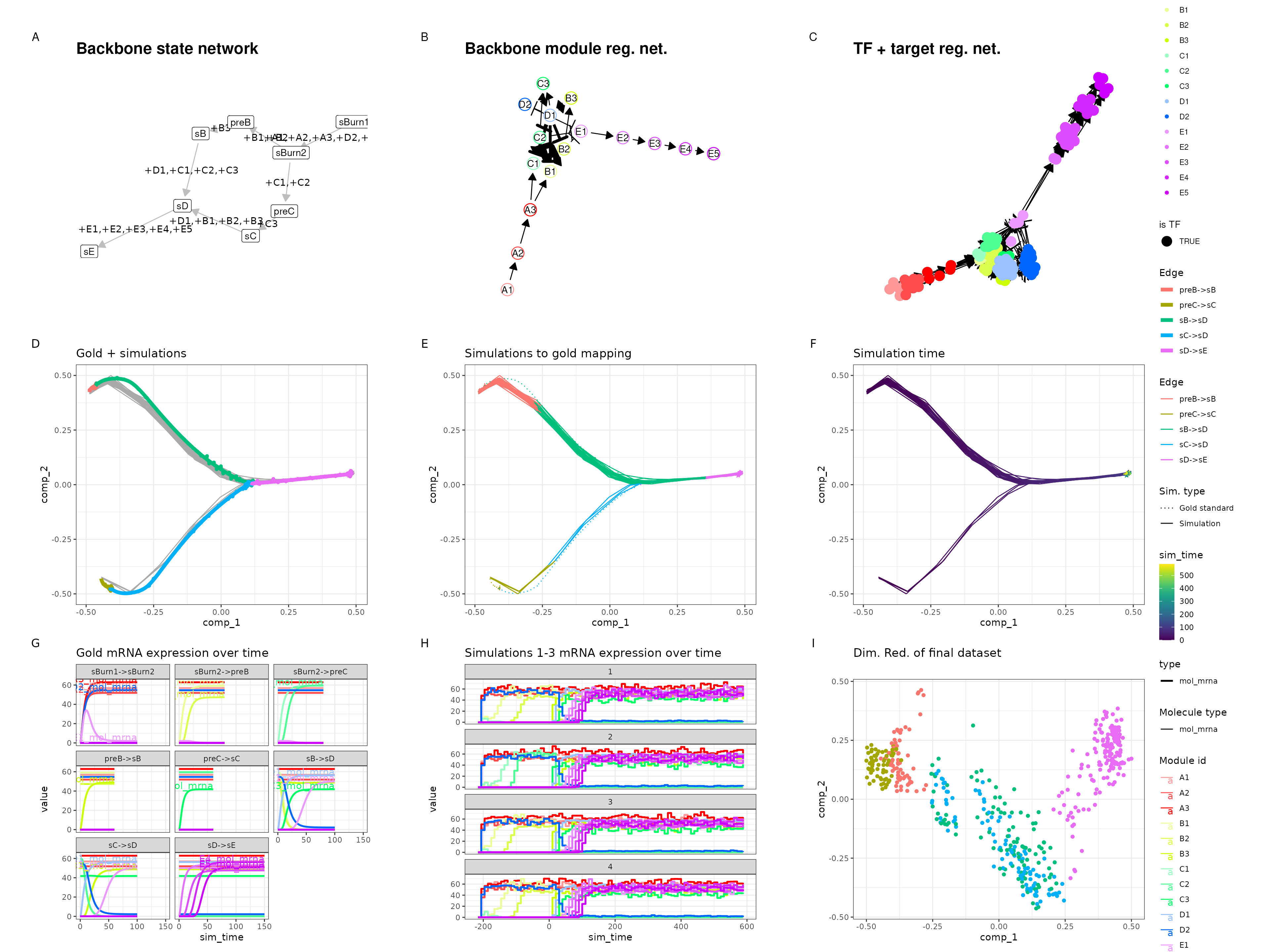## Cycle

set.seed(11)

backbone <- backbone_cycle()

init <- initialise_model(
backbone = backbone,
num_cells = 500,
num_tfs = 100,
num_targets = 0,
num_hks = 0,
simulation_params = simulation_default(census_interval = 10, ssa_algorithm = ssa_etl(tau = 300 / 3600)),
verbose = FALSE
)
out <- generate_dataset(init, make_plots = TRUE)
out$plot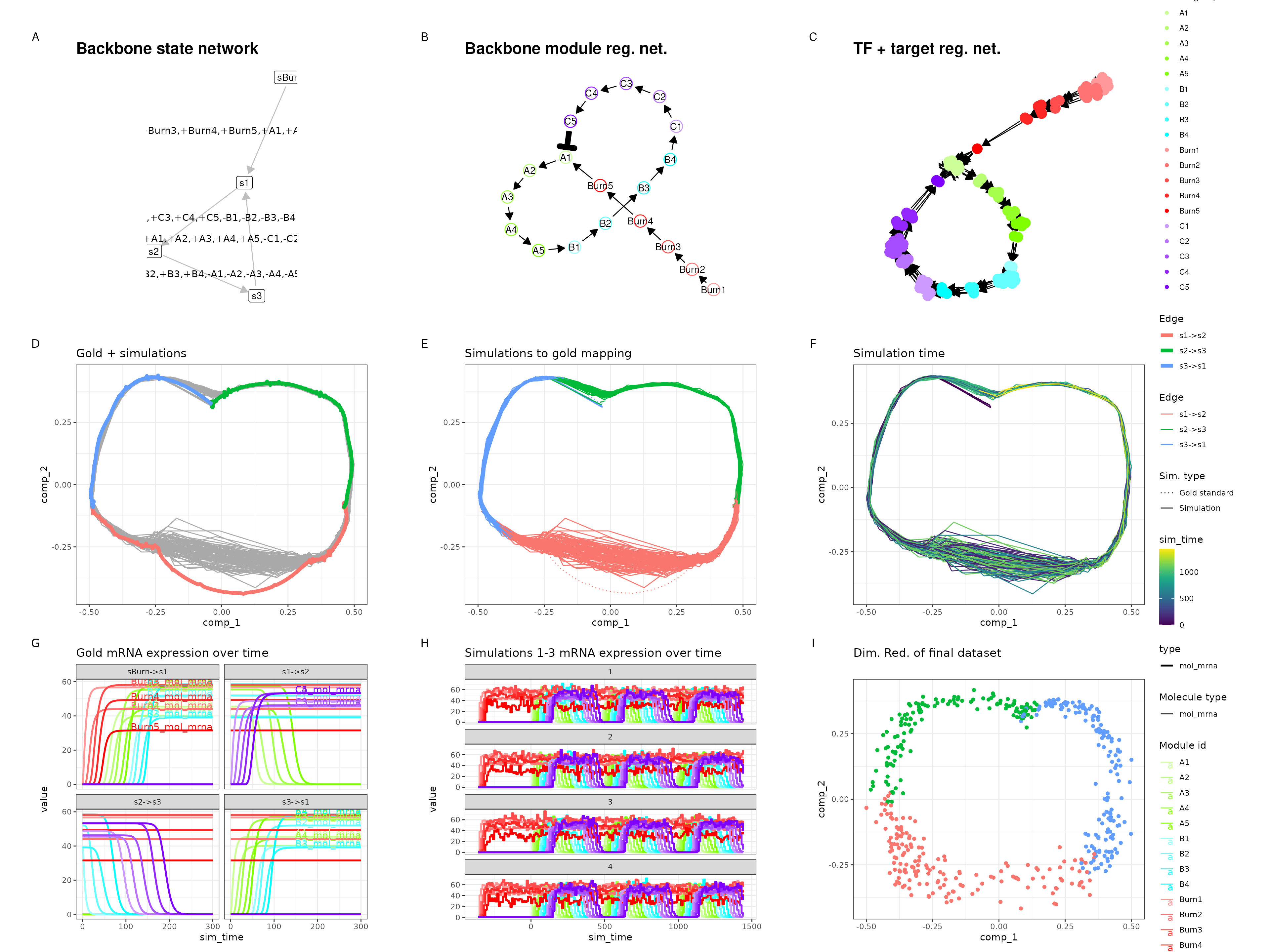## Disconnected set.seed(12) backbone <- backbone_disconnected() init <- initialise_model( backbone = backbone, num_cells = 500, num_tfs = 100, num_targets = 0, num_hks = 0, simulation_params = simulation_default(census_interval = 10, ssa_algorithm = ssa_etl(tau = 300 / 3600)), verbose = FALSE ) out <- generate_dataset(init, make_plots = TRUE) #> Warning in .generate_cells_predict_state(model): Simulation does not contain all #> gold standard edges. This simulation likely suffers from bad kinetics; choose a #> different seed and rerun. #> Warning in params$fun(network = network, sim_meta = sim_meta, params = model$experiment_params, : Certain backbone segments are not covered by any of the simulations. If this is intentional, please ignore this warning. #> Otherwise, increase the number of simulations (see ?simulation_default) or decreasing the census interval (see ?simulation_default). #> Warning in params$fun(network = network, sim_meta = sim_meta, params = model$experiment_params, : One of the branches did not obtain enough simulation steps. #> Increase the number of simulations (see ?simulation_default) or decreasing the census interval (see ?simulation_default). out$plot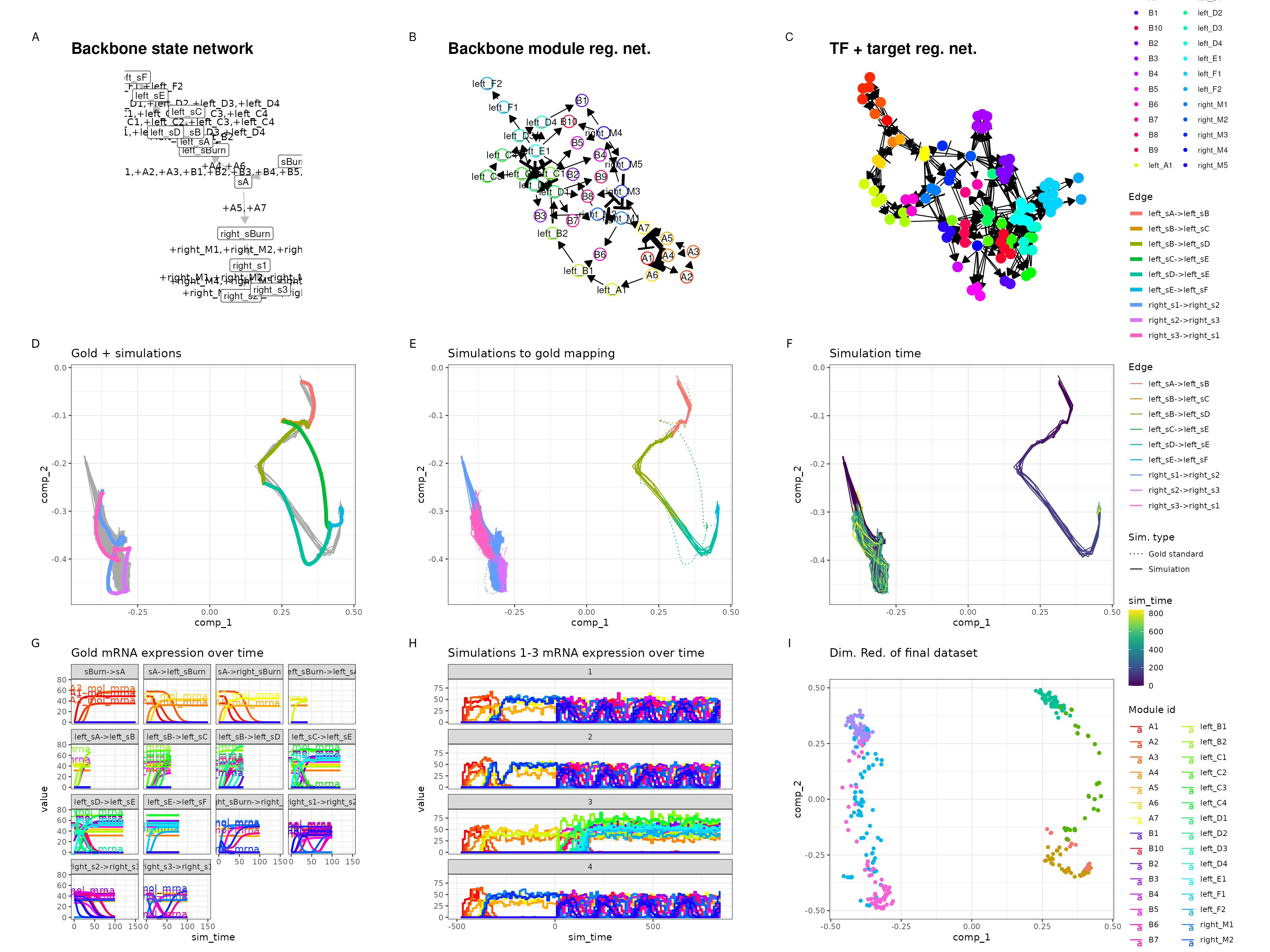## Linear simple

set.seed(13)

backbone <- backbone_linear_simple()

init <- initialise_model(
backbone = backbone,
num_cells = 500,
num_tfs = 100,
num_targets = 0,
num_hks = 0,
verbose = FALSE
)
out <- generate_dataset(init, make_plots = TRUE)
out$plot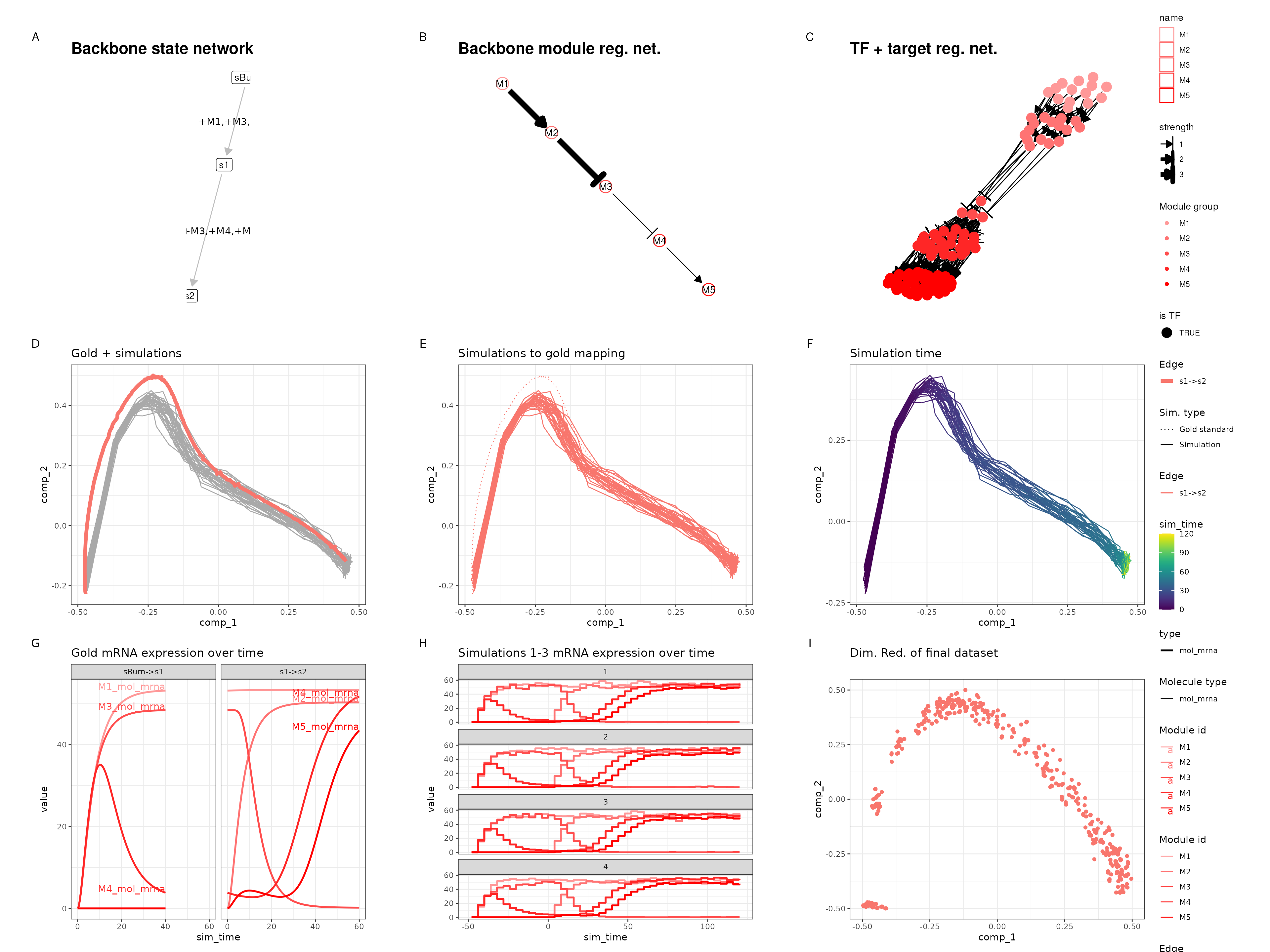## Cycle simple set.seed(14) backbone <- backbone_cycle_simple() init <- initialise_model( backbone = backbone, num_cells = 500, num_tfs = 100, num_targets = 0, num_hks = 0, verbose = FALSE ) out <- generate_dataset(init, make_plots = TRUE) out$plot